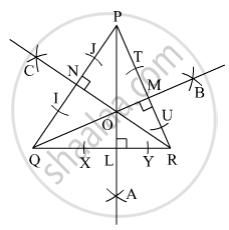SSC (English Medium) Class 8Maharashtra State Board
Share

# Draw an Acute Angled Pqr. Draw All of Its Altitudes. Name the Point of Concurrence as ‘O’. - SSC (English Medium) Class 8 - Mathematics

ConceptAltitudes of a Triangle

#### Question

Draw an acute angled Δ PQR. Draw all of its altitudes. Name the point of concurrence as ‘O’.

#### SolutionSteps of construction :
(i) Draw any acute angled ∆PQR.
(ii) With P as centre, draw an arc that cut the side QR at X and Y.
(iii) With X as centre and radius more than half of XY, draw an arc below QR. With Y as centre and same radius draw another arc that cut the previous arc at A.
(iv) Join PA that intersect QR at L. So, PL is the altitude on side QR.
In the same manner, draw QM⊥PR and RN⊥PQ.
Hence, ∆PQR is the required triangle with altitudes PL, QM and RN on sides QR, RP and PQ respectively with O as the point of concurrence of all the three altitudes.

Is there an error in this question or solution?

#### APPEARS IN

Balbharati Solution for Balbharati Class 8 Mathematics (2019 to Current)
Chapter 4: Altitudes and Medians of a triangle
Practice Set 4.1 | Q: 2 | Page no. 22
Solution Draw an Acute Angled Pqr. Draw All of Its Altitudes. Name the Point of Concurrence as ‘O’. Concept: Altitudes of a Triangle.
S